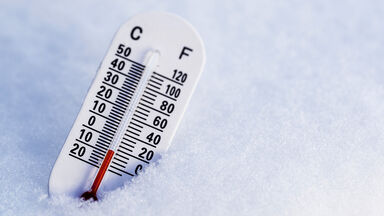# What's the Easiest Way to Convert Fahrenheit to Celsius?

What's the difference between Celsius and Fahrenheit? It's really a quirk of history and a simple mathematical conversion. Learn the easiest way to convert Fahrenheit to Celsius as well as the reverse calculation with simple equations and a chart.thermometer in snow Celsius and Fahrenheit

## Converting Fahrenheit to Celsius

Some would consider a quick estimate the easiest way to convert a Fahrenheit temperature to a Celsius temperature. Others want a more precise answer quickly. Here, you can learn both methods.

### Fahrenheit to Celsius Estimate Formula

Use this formula to get a good estimate of the Celsius temperature when converting from Fahrenheit. In this case / means divided by or to use division.

(Fahrenheit temp. [F]-30)/2 = Celsius temp.

In other words, if you’d like to convert a Fahrenheit temperature to an approximate Celsius temperature:

2. Subtract 30 from this figure (e.g., 100 - 30 = 70).
3. Divide your answer by 2 (e.g., 70 / 2 = 35).

### Fahrenheit to Celsius Exact Formula

If you want a more precise calculation, you can use the more exact formula. Using this calculation, we determine that 100 degrees Fahrenheit is equivalent to 37.78 degrees Celsius.

(F - 32) / 1.8 = C

In other words, if you'd like to convert a temperature reading in Fahrenheit to Celsius:

2. Subtract 32 from this figure (e.g., 100 - 32 = 68).
3. Divide your answer by 1.8 (e.g., 68 / 1.8 = 37.78)

### Converting Celsius to Fahrenheit

To convert Celsius to Fahrenheit, simply reverse the equation. In this case * means times, or to use multiplication.

(C * 1.8) + 32 = F

You can use this equation to show that 100 degrees Celsius is equal to 212 degrees Fahrenheit.

2. Multiply this figure by 1.8 (e.g., 100 * 1.8 = 180).
3. Add 32 to this figure (e.g., 180 + 32 = 212).

## Fahrenheit to Celsius Conversion Chart

If you really want an easy way to convert temperatures, you can use this Fahrenheit to Celsius conversion chart where the math has already been done for you.

## What's the Difference Between Fahrenheit and Celsius?

If you're from the United States, you're probably accustomed to describing temperature by Fahrenheit. A very hot summer day is 100 degrees, a pleasant spring morning is 50 degrees, and 0 degrees is ungodly cold. If you're from almost anywhere else, you likely favor Celsius, where 0 degrees is only pretty cold, 50 degrees is unspeakably hot, and 100 degrees happens on a stove, not outside.

### Are Fahrenheit and Celsius Readings Ever the Same?

If you were wondering if there is a temperature where Fahrenheit and Celsius are the same, it's at 40 below zero, also known as -40 or negative 40. At all other temperatures, the difference is history.

## History of Fahrenheit and Celsius Scales

Globally, the standard scale for everyday use is Celsius. Only the United States, islands freely associated with the US (Palau, the Marshall Islands and Micronesia), the Bahamas, the Caymans, and Liberia use Fahrenheit as their primary temperature measurement. Everybody else goes with Celsius.

### History of the Fahrenheit Scale

The Fahrenheit scale was created by Daniel Gabriel Fahrenheit in 1724. Fahrenheit invented his scale for use with mercury thermometers, which he also invented. The Fahrenheit scale is a bit older than the Celsius scale.

### History of the Celsius Scale

The Celsius scale was created by Anders Celsius in 1745, a couple decades after Fahrenheit. Curiously, Celsius created the reverse of the modern scale, treating 0 as the boiling point of water and 100 as the freezing point of water. Other scientists, notably Carl Linnaeus, flipped it later that year, making 0 the freezing point and 100 the boiling point of water.

Since it was originally built as a scale between 0 and 100, Celsius is also called centigrade. The word "centigrade" literally describes something that consists of or is divided into 100 degrees. That said, Celsius has been the preferred nomenclature since 1948.

## The Kelvin Temperature Unit

You may have heard of temperature being measured according to kelvin. Indeed, if you're involved in the sciences, you probably use kelvin. Kelvin is a bit different than Fahrenheit and Celsius. Celsius and Fahrenheit are both temperature scales, measuring temperatures in degrees.

### History of the Kelvin Unit

Named after Lord Kelvin, a kelvin is a standard scientific unit, like a kilogram for weight or a meter for distance. The proper way to use the word, then, is not "100 degrees Kelvin" but "100 kelvin." (Note the lower case "k" in kelvin.)

1 kelvin equals 1/273.16th of the triple point of water: that is, the point at which liquid water, water vapor and solid ice can coexist. In other words, 273.16 kelvin is the exact triple point of water.

### The Importance of Kelvin

The importance of kelvin is that it measures absolute temperature: absolute zero is 0 kelvin, the temperature at which all atomic motion (which creates heat) stops. There is no thermal energy whatsoever at absolute zero.

### Kelvin Conversion Formulas

Kelvin is primarily of value to scientists, since it simplifies calculation at extreme temperatures. For your convenience, the conversion equations follow.

• To convert: Celsius to kelvin: C + 273.15 = K.
• To convert Fahrenheit to kelvin: [(F - 32) / 1.8] + 273.15 = K

You convert Celsius to Kelvin by simply adding 273.15. If the outside temperature is 10 degrees Celsius, then it is 283.15 kelvin. That's easy enough.

Fahrenheit is a bit trickier. Take your degrees Fahrenheit, subtract 32, divide by 1.8, and finally add 273.15. Here's a quick example:

1. Say it is 100 degrees Fahrenheit outside.
2. Subtract 32 from 100 to get 68.
3. Divide 68 by 1.8 to get 37.78.
4. Add 273.15 to get 310.93. Thus, 100 degrees Fahrenheit is equal to 310.93 kelvin.